# Differentiability Of Some Basic Functions

Go back to  'LCD'

We will consider some examples to make this discussion more clear.

(i) $$\boxed{f\left( x \right) = {x^2}}$$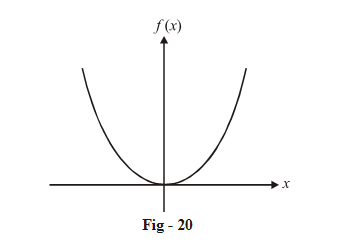The graph of f(x) is ‘smooth’. Lets verify this by evaluating the LHD and RHD at any general  x-coordinate.

\begin{align}{\rm{LHD}} &= \mathop {\lim }\limits_{h \to 0} \frac{{f\left( {x - h} \right) - f\left( x \right)}}{{ - h}}\\ &= \mathop {\lim }\limits_{h \to 0} \frac{{{{\left( {x - h} \right)}^2} - {x^2}}}{{ - h}}\\& = \mathop {\lim }\limits_{h \to 0} \frac{{{x^2} + {h^2} - 2xh - {x^2}}}{{ - h}}\\ &= \mathop {\lim }\limits_{h \to 0} \frac{{{h^2} - 2xh}}{{ - h}}\\ &= 2x\end{align}

\begin{align}{\rm{RHD}}&= \mathop {\lim }\limits_{h \to 0} \frac{{f\left( {x + h} \right) - f\left( x \right)}}{h}\\&= \mathop {\lim }\limits_{h \to 0} \frac{{{{\left( {x + h} \right)}^2} - {x^2}}}{h}\\&= \mathop {\lim }\limits_{h \to 0} \frac{{2xh + {h^2}}}{h}\\ &= 2x\end{align}

We see that LHD = RHD for every x value. Hence f(x) is everywhere differentiable (smooth).

Also the slope at any coordinate x has a numerical value 2x (equal to the LHD and RHD).

We say that the derivate of  $$f\left( x \right) = {x^2}$$ is 2x.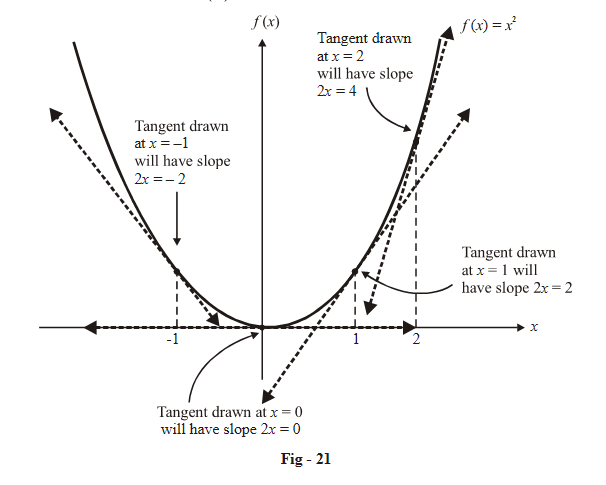(ii) $$\boxed{f\left( x \right) = \left| x \right|}$$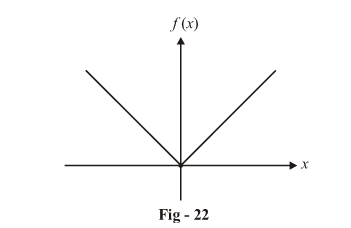The graph has a sharp turn (corner) at x = 0. This means that at x = 0, $$f\left( x \right) = \left| x \right|$$  should be non-differentiable. Lets verify this again :

\begin{align}&{\rm{LHD}} = \mathop {\lim }\limits_{h \to 0} \frac{{f\left( {x - h} \right) - f\left( x \right)}}{{ - h}}\\&\qquad {\rm{ }}\left. { = \mathop {\lim }\limits_{h \to 0} \frac{{f\left( {0 - h} \right) - f\left( 0 \right)}}{{ - h}}\,\,\,} \right\}\,\,\,{\rm{we}}\,{\rm{want}}\,{\rm{to}}\,{\rm{evaluate}}\,{\rm{LHD}}\,{\rm{at}}\,x\,{\rm{ = }}\,{\rm{0}}\\&\qquad{\rm{ }} = \mathop {\lim }\limits_{h \to 0} \frac{{\left| { - h} \right|}}{{ - h}}\\ &\qquad= {\rm{ }}-{\rm{ }}1\end{align}

Similarly,

RHD = 1

This result is visually obvious from Fig - 22; the left segment is  $$y = - x$$ which has a slope –1, while the right segment is $$y = x,$$  which has slope 1.

(iii)    $$\boxed{f\left( x \right) = \left[ x \right]\,\,{\text{and}}\,\,f\left( x \right) = \left\{ x \right\}}$$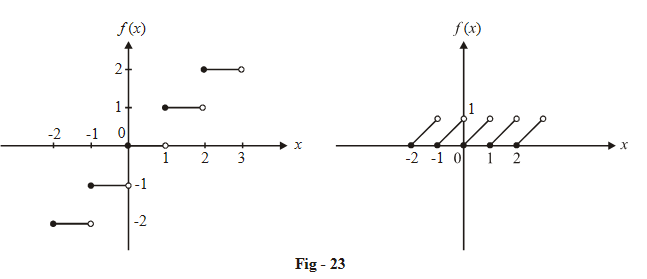Both these functions are discontinuous, so let us carefully understand how the derivatives will be evaluated.

$$f(x){\rm{ }} = {\rm{ }}[x]$$

\begin{align}&LHD = \mathop {\lim }\limits_{x \to {I^{\;{\bf{-}}}}} \frac{{[x] - I}}{{x - I}}\\&\quad\quad{\rm{ }} = \mathop {\lim }\limits_{h \to 0} \frac{{[I - h] - I}}{{ - h}} = \mathop {\lim }\limits_{h \to 0} \frac{1}{h} = \infty \\&RHD = \mathop {\lim }\limits_{h \to 0} \frac{{[I + h] - I}}{h} = \mathop {\lim }\limits_{h \to 0} \frac{0}{h} = 0\end{align}

We see that the LHD is infinity, since there is a finite change in the function’s value in going from I –  to I, i.e., there is a finite change over an infinitesmally small interval.

$$f\left( x \right) = \left\{ x \right\}$$

\begin{align}&LHD = \mathop {\lim }\limits_{x \to I{\;^ - }} \frac{{\left\{ x \right\} - 0}}{{x - I}}\\&\quad\quad{\rm{ }} = \mathop {\lim }\limits_{h \to 0} \frac{{\left\{ {I - h} \right\} - 0}}{{ - h}} = \mathop {\lim }\limits_{h \to 0} \frac{1}{{ - h}} = - \infty \\ &RHD = \mathop {\lim }\limits_{h \to 0} \frac{{\left\{ {I + h} \right\} - 0}}{h} = \mathop {\lim }\limits_{h \to 0} \frac{h}{{ - h}} = 1 \end{align}

We see that the LHD is – infinity, since there is a finite (negative) change in the function’s value in going from $${{\rm{I}}^ - }{\rm{ }}$$ to $$I$$.

Thus, f(x) is non-differentiable at all integers. At all other values of x, f(x) is differentiable with the derivative’s value being 1.

Derivative of discontinuous functions will become more clear when you study impulse (Dirac delta) functions at the undergraduate level.

(iv)   $$\boxed{f\left( x \right) = \sin x}$$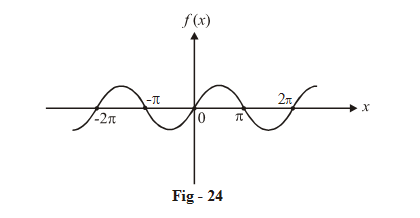This function seems ‘smooth’ everywhere. We will now verify this:

\begin{align}&{\rm{LHD}}\; = \mathop {\lim }\limits_{h \to 0} \frac{{\sin \left( {x - h} \right) - \sin x}}{{ - h}}\\&\qquad{\rm{ \; }} = \mathop {\lim }\limits_{h \to 0} \frac{{2\cos \left( {x - \frac{h}{2}} \right)\sin \left( { - \frac{h}{2}} \right)}}{{ - h}}\\&\qquad\; = {\rm{}}cosx\\&{\rm{RHD}} = \mathop {\lim }\limits_{h \to 0} \frac{{sin\left( {x + h} \right) - \sin x}}{h}\\&\qquad{\rm{ }} = \mathop {\lim }\limits_{h \to 0} \frac{{2\cos \left( {x + \frac{h}{2}} \right)\sin \left( {\frac{h}{2}} \right)}}{h}\\&\quad\quad{\rm{ }} = {\rm{ }}cosx \end{align}

Hence, LHD = RHD for all values of x; sin x is everywhere differentiable. The derivative at x (slope of tangent at x) has the numerical value cos x.

$$\Rightarrow$$ The derivative of sin x is cos x.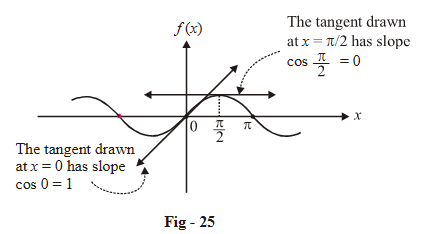By now you should be pretty clear about the meaning and graphical significance of differentiability and evaluating the derivative. Evaluating the derivative using the process we have described above is called differentiation using first principles.

This discussion is extremely important to whatever we’ll study afterwards. You must ensure that you’ve fully understand this.

Limits
grade 11 | Questions Set 1
Limits
Limits
grade 11 | Questions Set 2
Limits